Most Affordable JEE | NEET | 8,9,10 Preparation by Kota's Top IITian Doctor Faculties

# Electricity Class 10 NCERT Notes

Class 10`

Welcome to the comprehensive guide on Electricity Class 10 NCERT Notes . In this article, we will cover all the important topics related to electricity as per the NCERT syllabus. Instead of just passively reading the notes, we encourage you to actively engage with the content and practice the concepts to gain a deeper understanding of this fundamental topic in physics. So, let's dive in and explore the world of electricity

Electricity: Electric current, electric circuit, voltage or electric potential, resistance and (Ohm’s law).

Electric Current: The flow of electric charge is known as Electric Current, Electric current is carried by moving electrons through a conductor.
By convention, electric current flows in the opposite direction to the movement of electrons.

Electric Circuit: Electric circuit is a continuous and closed path of electric current.

Expression of Electric Current: Electric current is denoted by the letter ‘I’. Electric current is expressed by the rate of flow of electric charges. Rate of flow means, the amount of charge flowing through a particular area in unit time.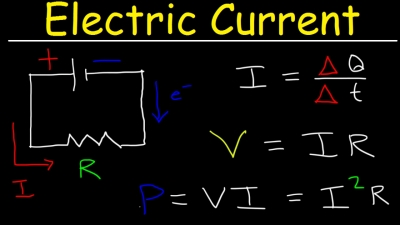If a net electric charge (Q) flows through a cross-section of a conductor in time t, then,

Where I is electric current, Q is a net charge and t is a time in second.

S.I. Unit of Electric Charge and Current: S.I. unit of electric charge is coulomb (C).
One coulomb is nearly equal to 6 × 1018 electrons. S.I. unit of electric current is ampere (A). Ampere is the flow of electric charge through a surface at the rate of one coulomb per second. This means, if 1 coulomb of electric charge flows through a cross section for 1 second, it would be equal to 1 ampere.
Therefore, 1 A = 1 C/1 s

Small Quantity of Electric Current: Small quantity of electric current is expressed in milliampere and microampere. Milliampere is written as mA and microampere as pA.
1 mA (milliampere) = 10-3 A
1 pA (microampere) = 10-6 A

Ammeter: An apparatus to measure electric current in a circuit.,

Charge: Like mass, the charge is the fundamental property of matter. There are two types of charge
(i) Positive charge.
(ii) Negative charge.

Positive and Negative Charge:

When you rub a glass rod with silk, it acquires a positive charge, and when you rub an ebonite rod with wool, it acquires a negative charge. These charges are known as positive and negative charges, respectively, and are fundamental to the study of electricity.

Two important properties of electric charge are: (i) Opposite charges attract each other, and like charges repel each other. (ii) The force between two charges is directly proportional to the product of the charges and inversely proportional to the square of the distance between them.

The SI unit of electric charge is coulomb (C), which is defined as the quantity of charge that flows through a circuit when one ampere of current flows through it for one second. Therefore, 1C = 1A × 1s.

Electric potential and potential difference are other important concepts in electricity.

Electric Potential: The amount of electric potential energy at a point is called electric potential.
Potential Difference:

Electric potential difference is the difference in the amount of electric potential energy between two points in an electric circuit. This is commonly known as voltage, which is the amount of work required to move a unit charge between two points against a static electric field. The formula for voltage is V = W/Q, where V is voltage, W is work done, and Q is charge.

The SI unit of electric potential difference or voltage is the volt (V), named in honor of the Italian physicist Alessandro Volta. One volt of electric potential difference is equal to one joule of work done to move a charge of one coulomb from one point to another in an electric circuit. Therefore, 1V = 1Joule/1Coulomb = 1J/1C, where J is the unit of work and C is the unit of charge.

Voltmeter: An apparatus to measure the potential difference or electric potential difference between two points in an electric circuit.

Galvanometer: It is a device to detect current in an electric circuit.

### Ohm’s Law - Electricity Class 10 NCERT Notes

According to Ohm’s Law, the electric potential difference between two points in an electric circuit is directly proportional to the electric current, when the temperature is constant. This means that the potential difference V varies directly as electric current, and can be expressed mathematically as V ∝ I. Additionally, the equation V = RI relates potential difference (V) to current (I), where R is a constant value known as resistance, which is unique to the given conductor and temperature.

Using Ohm's law, we can also express the relationship between resistance, voltage and current as follows: I = V/R and R = V/I.

Resistance: Resistance is the property of conductor which resists the flow of electric current through it.
S.I. unit of resistance is ohm. Ohm is denoted by Greek letter ‘Q’

1 Ohm:

In an electric circuit, 1 ohm (Ω) of resistance (R) is equivalent to a flow of 1A of electric current between two points with a potential difference of 1V. This can be expressed mathematically as 1Ω = 1V/1A.

According to Ohm’s Law, the electric current through a resistor is inversely proportional to its resistance. Therefore, an increase in resistance leads to a decrease in electric current, and vice versa. The graph of potential difference (V) versus electric current (I) is always a straight line.

To determine the potential difference (V), we can use Ohm's Law, which states that V = IR. For example, if the resistance is 15Ω and the current is 15A, then the potential difference can be calculated as follows:

V = IR = 15Ω x 15A = 225V.

Resistance: Resistance is a property of conductor due to which it resists the flow of electric current through it. A component that is used to resist the flow of electric current in a circuit is called a resistor.
In practical application, resistors are used to increase or decrease the electric current.

Variable Resistance: The component of an electric circuit which is used to regulate the current, without changing the voltage from the source, is called variable resistance.

Rheostat: This is a device which is used in a circuit to provide variable resistance.

Cause of Resistance in a Conductor - Electricity Class 10 NCERT Notes

The flow of electrons in a conductor constitutes electric current. The hindrance to the flow of electrons caused by the attraction between the positive particles of the conductor is known as resistance.

Several factors influence the resistance of a conductor, including the nature of the material, the length, and the area of cross section of the conductor.

(i) Nature of Material: Some materials are good conductors and offer the least resistance, while others are bad conductors or insulators and impede the flow of electric current.

(ii) Length of Conductor: Resistance is directly proportional to the length of the conductor. This implies that resistance increases with an increase in the length of the conductor, making long electric wires more resistant to electric current.

(iii) Area of Cross Section: Resistance is inversely proportional to the area of cross section of the conductor. This means that resistance decreases with an increase in the area of the conductor. A larger area of the conductor facilitates the flow of electric current and thus decreases resistance.

The resistance (R) of a conductor is given by the equation R = ρ l / A, where ρ (rho) is the electrical resistivity of the material of the conductor. The resistivity is a proportionality constant that relates the resistance of a conductor to its length and area of cross section. The SI unit of resistivity is ohm-meter (Ωm).

### Resistivity - Electricity Class 10 NCERT Notes

The resistance offered by a cube of material of side 1m when current flows perpendicular to its opposite faces is known as resistivity. Its S.I. unit is ohm-meter (Ωm). We can define resistivity as ρ = RAl, and it is also known as specific resistance. The nature of the material of the conductor determines its resistivity. Materials with resistivity in the range of 10-8 Ωm to 10-6 Ωm are considered very good conductors, such as silver with a resistivity of 1.60 × 10-8 Ωm and copper with a resistivity of 1.62 × 10-8 Ωm. Rubber and glass are very good insulators with resistivity in the order of 10-12 Ωm to 10-8 Ωm. Additionally, the resistivity of materials varies with temperature.

Two common ways to combine resistors are through series combination and parallel combination.

1. Resistors in Series: When resistors are joined from end to end, it is called in series. In this case, the total resistance of the system is equal to the sum of the resistance of all the resistors in the system.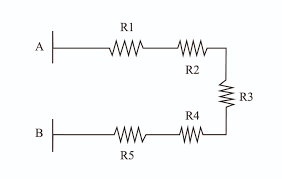Let, three resistors R1, R2, and R3 get connected in series.
Potential difference across A and B = V
Potential difference across R1, R2 and R3 = V1, V2 and V3
Current flowing through the combination = I
We, know that
V= V1 + V2 + V3 …. (i)
According to Ohm’s Law :
V1 = IR1, V2 = IR2 and V3 = IR3 ….. (ii)
Let, total resistance = Rs
Then, V = IRs …(iii)
From equations (i) and (ii) and (iii)
IRs = IR1 + IR2 + IR3
Rs = R1 + R2 + R3
When the resistors are connected in series, the current flowing through each resistor is the same and is equal to the total current.

2. Resistors in Parallel- Electricity Class 10 NCERT Notes

When we connect resistors in parallel, the sum of the reciprocal of the resistance of each resistor is equal to the reciprocal of the total resistance of the system.

Consider three resistors, R1, R2, and R3, connected in parallel. Let the potential difference across points A and B be V, and the total current flowing between points A and B be I. The currents flowing through resistors R1, R2, and R3 are I1, I2, and I3, respectively.

From equation (i), we know that the total current I is the sum of I1, I2, and I3. Since the potential difference across each resistor is the same, according to Ohm's Law:

In a parallel combination, the potential difference across each resistor is equal to the total potential difference. We can calculate the total current through the circuit by adding the electric current through individual resistors. For example, in a circuit with resistors of 6A, 48A, 30A, 12A, and 24A, the total current would be 120A

Heating Effect of Electric Current: When an electric current flows through a purely resistive conductor, the dissipation of electrical energy causes the resistor to get heated, and the energy of the electric current is entirely dissipated in the form of heat. This phenomenon is commonly known as the Heating Effect of Electric Current. For instance, the filament of an electric bulb gets heated when electric energy is supplied, causing it to emit light. The heating of the electric bulb occurs due to the heating effect of electric current.

Cause of Heating Effect of Electric Current:

The flow of electric current produces heat as it surpasses the resistance of the conductor. The amount of heat generated by the electric current is directly proportional to the resistance of the conductor. Therefore, the production of heat is an inevitable outcome of the flow of electric current through a conductor. Many appliances, including electric irons, electric heaters, and electric geysers, utilize this heating effect.

Joule’s Law Of Heating: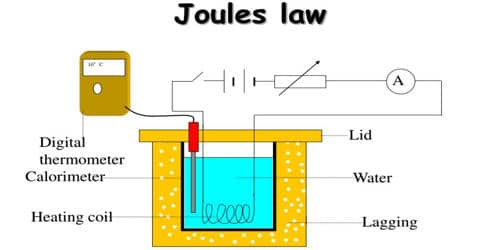To calculate the power input (P) in a circuit with an electric current (I) flowing through a resistor with resistance (R) and potential difference (V), the equation P = VI can be used. The heat produced (H) in time (t) can be calculated using the expression H = VIt, where V is the potential difference, I is the current, and t is the time. This is known as the Heating Effect of Electric Current and is used in appliances such as electric irons, heaters, and geysers.

Joule's Law of Heating states that the heat produced in a resistor is directly proportional to the square of the current flowing through it, directly proportional to the resistance for a given current, and directly proportional to the time for which the current is flowing through the resistor. This can be expressed as H = I2Rt, where H is the heat produced, I is the current, R is the resistance, and t is the time.

Electric Bulb: In an electric bulb, the filament of bulb gives light because of the heating effect of electricity. The filament of bulb is generally, made of tungsten metal, having melting point equal to 3380°C.

Electric Iron: The element of electric iron is made of alloys having high melting poir^ Electric heater and geyser work on the same mechanism.

Electric Fuse: Electric fuse is used to protect the electric appliances from high voltage if any. Electric fuse is made of metal or alloy of metals, such as aluminum, copper, iron, lead, etc. In the case of flow of higher voltage than specified, fuse wire melts and protect the electric appliances.
Fuse of 1A, 2A, 3A, 5A, 10A, etc., used for domestic purpose.
Suppose, if an electric heater consumes 1000W at 220 V.
Then electric current in circuit
I = PV
I = 1000W220V = 4.5 A
Thus, in this case of 5A should be used to protect the electric heater in the flow of higher voltage.

Electric Power
S.I. unit of electric power is watt (W).
1W = 1 volt × 1 ampere = 1V × 1A
I kilowatt or 1kW = 1000 W
Consumption of electricity (electric energy) is generally measured in kilowatt.
Unit of electric energy is kilowatt-hour (kWh).
1 kWh = 1000 watt × 1 hour = 1 unit = 1000 W × 3600 s
1 kWh = 3.6 x 106 watt second = 3.6 × 106 J

Conductor: The material which can allow the flow of electrons through itself is called the conductor. It has a large number of free electrons. It offers low opposition in the flow of current.

Insulator: The material which does not allow the flow of electrons through itself is called insulator. It has less or no free electrons. It offers high opposition in the flow of current.

Electric Current: The amount of flow charge through any cross-sectional area of a conductor in unity time is called Electric Current.
It is represented by ‘I’
I = QT

Unit of Electric Current: It is CS-1 (coulomb per second) or Ampere (A). Electric Current is a scalar quantity. It is measured by an ammeter.

Direction: The direction of conventional current (or practical current) is opposite to the flow of electrons.

Electric potential: Electric Potential at any point in the electric field is defined as the amount of work done to bring the unit positive charge from infinity (from outside the electric field) to that point.
V =WQ, S.I. unit of Electric Potential is JC-1 or volt (V). It is a scalar quantity. The +ve charge flows from higher to lower potential. The -ve charge flows from lower to a higher potential. The difference of electric potential between any two points in the electric field is called Electric Potential difference. It is known as a voltage which is equal to the work done per unit charge between two points against the static electric field.
VAB = VA – VB = WABQ
Electric Potential difference is measured by a voltmeter.

Ohm’s Law: According to this law “Under the constant physical condition the potential difference across the conductor is directly proportional to the current flowing through the conductor.”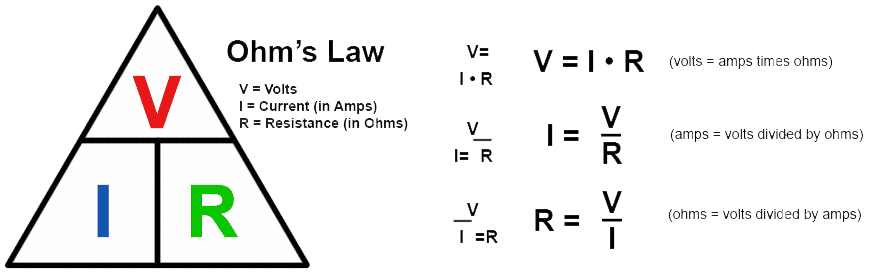V ∝ I
V = IR …[Where R is proportionality constant called resistance of conductor]
⇒ I = VR
R depends upon nature, geometry and physical condition of the conductor.

The heat generated by electric current: The potential difference between two points in an electrical field is equal to the work done in moving a unit charge from one point to another.
Then, work is done, W = VQ and Q = I × t
W = V × I × t
From Ohm’s Law, we know that
V = IR
W = IR × I × t = I2.Rt
Since heat produced by the electric current is equal to work done, W
H = W
⇒ H (heat) = I2Rt Joule.

Resistance: Ratio of the applied voltage to the current flowing in the conductor is called resistance of the conductor.
⇒ R = VI
S.I. Unit of resistance is VA-1 or ohm (Ω).
Resistance is the opposition offered by the conductor in the flow of current.
Practically it is
R ∝ L (L is the length of a conductor)
R ∝ 1/A (A is the area of a conductor)
So, R ∝ L/A
R = ρL/A …[Where p is proportionality constant called specific resistance of conductor
It only depend upon nature (material) and temperature of conductor.

Specific resistance or Resistivity = ρ = RA /L
It’s S.I. Unit is Qm

Combination of resistance:

• In this combination the current across every component is same but potential across every component is different.
• If resistance R1, R2 and R3 are connected in series with a battery of Potential V, then equivalence resistance of the combination
R = R1 + R2 + R3

The parallel combination of resistance - Electricity Class 10 NCERT Notes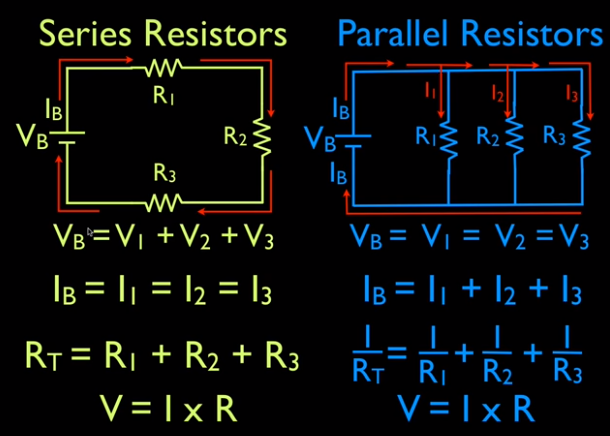• In this combination the current across every component is different. But potential across every component is the same.
• If resistance R1, R2 and R3 are connected in parallel with a battery of Potential V, then equivalence resistance of combination
1R=1R1+1R2+1R3

Electric Energy is amount of work done to maintain the continuous flow of electric current in the circuit.
Its S.I. unit is joule (J).

Electric power (P): The electric work done per unit time is called electric power.
Electric Power = ElectricworkdoneTimetaken
or P = Wt
Electric power is also defined as the electric energy consumed per unit time.
P = Et
S.I. unit of electric power is Watt. When one joule of energy is used for one second, electric power is equal to one watt.

Derivation of formula for electric power:
We know that electric work done, W = V × I × t or P = VItt
P = VI
Electric power in watts = Volts × ampere
Also V = IR …[According to Ohm’s Law]
So P = IR × I
P = I2R
We know that I = VR
P = (VR)2 × R = V2R Watt
The maximum value of electric current that can pass through an electric appliance without damaging electric appliance is called current rating of electric appliance.

Electricity Class 10 NCERT Notes

Download the eSaral App for complete Class 10 Video lectures, Study material, revision and much more.